## Dichroic Polynomial

A Polynomial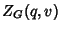in two variables for abstract Graphs. A Graph with one Vertex has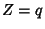. Adding a Vertex not attached by any Edges multiplies theby. Picking a particular Edge of a Graph, the Polynomial foris defined by adding the Polynomial of the Graph with that Edge deleted to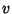times the Polynomial of the graph with that Edge collapsed to a point. Settinggives the number of distinct Vertex colorings of the Graph. The dichroic Polynomial of a Planar Graph can be expressed as the Square Bracket Polynomial of the corresponding Alternating Link bywhere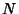is the number of Vertices in. Dichroic Polynomials for some simple Graphs are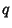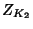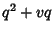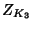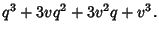References

Adams, C. C. The Knot Book: An Elementary Introduction to the Mathematical Theory of Knots. New York: W. H. Freeman, pp. 231-235, 1994.